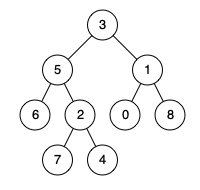Formatted question description: https://leetcode.ca/all/1650.html

# 1650. Lowest Common Ancestor of a Binary Tree III

Medium

## Description

Given two nodes of a binary tree p and q, return their lowest common ancestor (LCA).

Each node will have a reference to its parent node. The definition for Node is below:

class Node {
public int val;
public Node left;
public Node right;
public Node parent;
}


According to the definition of LCA on Wikipedia: “The lowest common ancestor of two nodes p and q in a tree T is the lowest node that has both p and q as descendants (where we allow a node to be a descendant of itself).”

Example 1:Input: root = [3,5,1,6,2,0,8,null,null,7,4], p = 5, q = 1

Output: 3

Explanation: The LCA of nodes 5 and 1 is 3.

Example 2:Input: root = [3,5,1,6,2,0,8,null,null,7,4], p = 5, q = 4

Output: 5

Explanation: The LCA of nodes 5 and 4 is 5 since a node can be a descendant of itself according to the LCA definition.

Example 3:

Input: root = [1,2], p = 1, q = 2

Output: 1

Constraints:

• The number of nodes in the tree is in the range [2, 10^5].
• -10^9 <= Node.val <= 10^9
• All Node.val are unique.
• p != q
• p and q exist in the tree.

## Solution

Use a set to store nodes. Start from p and obtain all the nodes from p to the root node using the nodes’ parents. Then start from q and move towards the root. If a node is already in the set, then the node is a common ancestor of p and q. The first common ancestor that is in the set is the lowest common ancestor of p and q.

/*
// Definition for a Node.
class Node {
public int val;
public Node left;
public Node right;
public Node parent;
};
*/

class Solution {
public Node lowestCommonAncestor(Node p, Node q) {
Set<Node> set = new HashSet<Node>();
Node temp = p;
while (temp != null) {
temp = temp.parent;
}
temp = q;
while (temp != null) {
if (set.contains(temp))
break;
else
temp = temp.parent;
}
return temp;
}
}